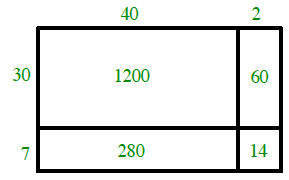### Home > CC1 > Chapter 2 > Lesson 2.3.1 > Problem2-55

2-55.

Draw a generic rectangle to multiply $37·42$. Homework Help ✎

1. Write an equation using addition that shows the total area of the generic rectangle.What are the smaller areas which combine to make the total area of the rectangle?

$1200+280+60+14=1554$

2. Multiply $37·42$ by using the standard algorithm for multiplication. That is, place one of the numbers under the other and use the procedure for multiplication that you were taught in previous courses. Show your work. Compare your result with your answer from part (a).

You should remember this method from previous math classes. To get you started, here is the correct set-up:

$\begin{array}\quad \ \ \ 42\\\times37\\ \hline\end{array}$

When you complete this problem, you should have the same answer as in part (a).

3. Which method of multiplication do you prefer? Why?

The answer depends on which method you like better. Write your response with complete sentences and justify it.